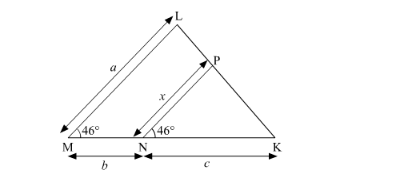# In the given figure, LM = LN = 46°.

Question:

In the given figure, LM = LN = 46°. Express x in terms of aand c where abc are lengths of LM, MN and NK respectively.Solution:

Given: In the given figure $\angle \mathrm{LMN}=\angle \mathrm{PNK}=46^{\circ}$

TO EXPRESS: x in terms of abc where a, b, and c are the lengths of LM, MN and NK respectively.

Here we can see that $\angle \mathrm{LMN}=\angle \mathrm{PNK}=46^{\circ}$. It forms a pair of corresponding angles.

Hence, LM || PN

In ∆LMK and ∆PNK,

∠LMK=∠PNK          Corresponding angles∠LKM=∠PKN          Common∴∆LMK~∆PNK       AA Similarity

MLNP=MKNKax=b+ccx=acb+c

Hence we got the result as $x=\frac{a c}{b+c}$.